• keywords：Word, Pseudocode 三种方式： 1.latex 需要安装，宏包导入不对或者冲突的话，会导致莫名其妙的报错，debug的时候一头雾水。 2.Aurora 易用性介于两者之间...哪位同学知道怎么用overleaf插入word请赐教，毕
keywords：Word, Pseudocode
三种方式： 1.latex 需要安装，宏包导入不对或者冲突的话，会导致莫名其妙的报错，debug的时候一头雾水。 2.Aurora 易用性介于两者之间，可直接作为插件在word文档编译，推荐使用。 3.overleaf 在线编译最方便 附上链接：https://cn.overleaf.com/
注1：其中前两个可插入到word文档（可编辑），使用overleaf只能生成pdf，自行转换吧。哪位同学知道怎么用overleaf插入到word请赐教，毕竟在线编辑还是最方便的嘛（懒汉的心声哈哈） 注2：前些年的网上代码大多不可用，大家不用费劲调试了。 挖个坑 争取月底填
终于准备写一些东西了，不准备做技术向博主，想到什么写什么吧，权当记录生活。像工程开发、职业生活、异构无线网络垂直切换方向的研究探讨、健身、打球、旅行、情感、杂记等等吧。争取至少周更吧。
第一次写就不讲究排版了，希望能陪我见证自己的成长。
博客消息可能不能及时看到，留个微信吧：cqu1995
2020/10/11
展开全文ieee论文
• 最近论文中要插入伪代码，网上主流的方法都是Aurora，但自己安装的时候遇到各种问题。。。这个古老的插件实在是太麻烦了。 正常安装的话应该是只支持32位的Office（这年头了谁还用32位啊）。 操作系统：Win10 ...
最近论文中要插入伪代码，网上主流的方法都是Aurora，但自己安装的时候遇到各种问题。。。这个古老的插件实在是太麻烦了。 正常安装的话应该是只支持32位的Office（这年头了谁还用32位啊）。

操作系统：Win10 Office版本：2019, 64位

需要安装Aurora+MikTex2.9，下载地址
1 安装
先安装MiKTeX 2.9然后安装Aurora，安装时不要选miktex再运行keygen进行破解
（网上好多教程说把时间改成2009年或2005年，但自己Office改时间后就会出问题，所以自己没这么设置） 自己都没有安装在默认路径，而是装在了D盘，事实证明不影响使用。
2 设置
设置paths，找到自己安装路径中相应文件填入。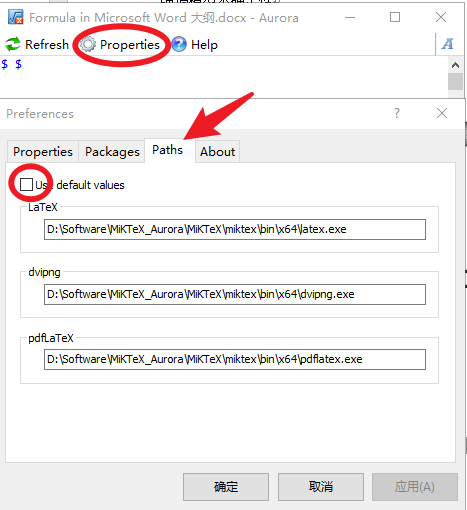这时就可以写一些简单的代码了。比如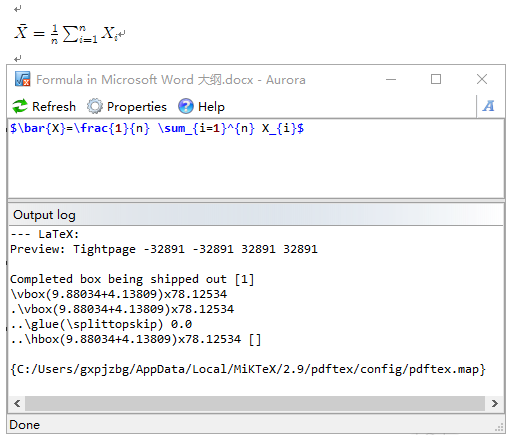3 伪代码配置
伪代码需要安装额外的包，网上常见的说法是在 Packages 中输入，但自己一直报错说

problems running latex

3.1 设置 Rendering method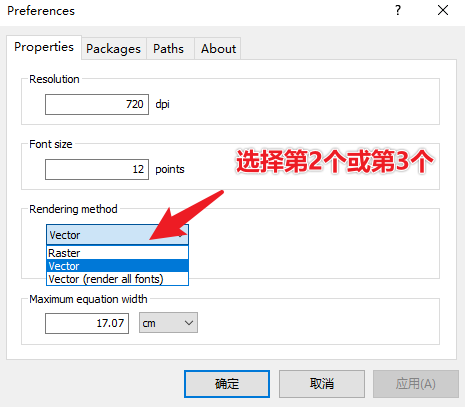3.2 【重要】安装包！！！ 自己一开始安装网上教程一直不成功的原因就是这里。 管理员身份运行 miktex-console.exe ，自己的位置在

D:\Software\MiKTeX_Aurora\MiKTeX\miktex\bin\x64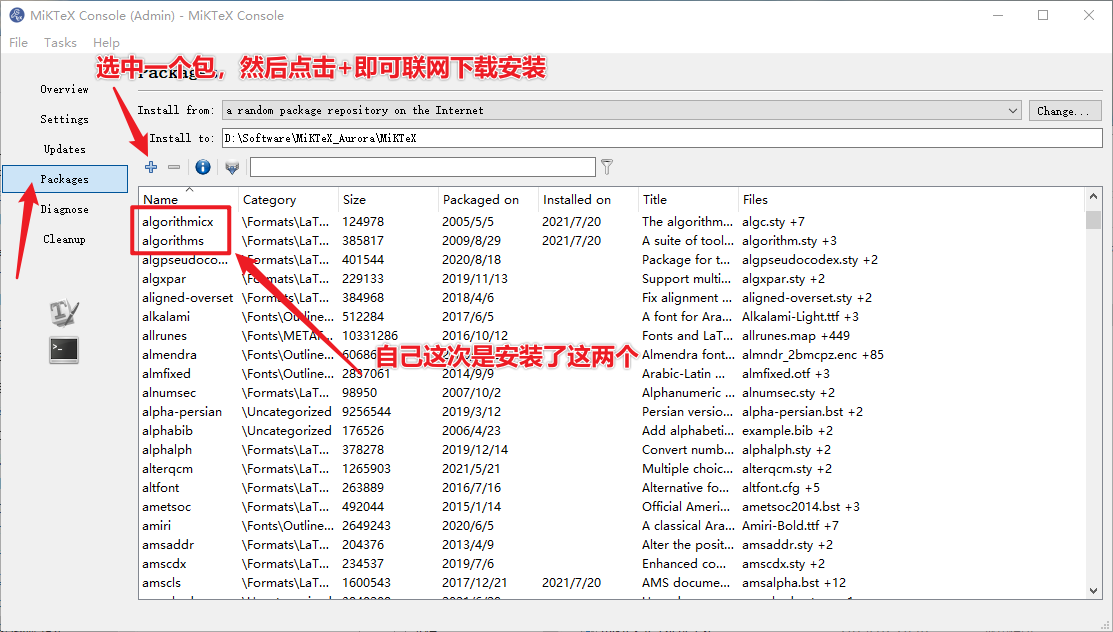3.3 添加包 这个网上有很多示例了，差别不大。下面摘录几个，备用。 自己用的是第二个。第一个的包看上去更多一些，但常见的代码用第二个就够用了。 如果用第一个的话还是会报错 problems running latex，应该是还需要下载别的库，自己就先不折腾了。
\usepackage{amsmath}
\usepackage{amssymb}
% \usepackage{euler}
\providecommand{\abs}{\left\lvert#1\right\rvert}
\providecommand{\norm}{\left\lVert#1\right\rVert}
\usepackage{bbm}
\usepackage{CJK}
\usepackage{listings}
\usepackage{xcolor}
\usepackage{listings}
\usepackage{amsmath,bm,graphicx,multirow,bm,bbm,amssymb,psfrag,algorithm,subfigure,color,mdframed,wasysym,subeqnarray,multicol}

\usepackage{algorithm}
\usepackage{algpseudocode}
\usepackage{amsmath}
\renewcommand{\algorithmicrequire}{\textbf{Input:}}
\renewcommand{\algorithmicensure}{\textbf{Output:}}


\documentclass{article}

\usepackage{multirow}
\usepackage{algorithm}
\usepackage{algpseudocode}
\usepackage{amsmath}
\usepackage{geometry}
\usepackage{algorithmicx}
\usepackage{algpseudocode}

\renewcommand{\algorithmicrequire}{\textbf{Input:}} % Use Input in the format of Algorithm
\renewcommand{\algorithmicensure}{\textbf{Output:}}

4 编写伪代码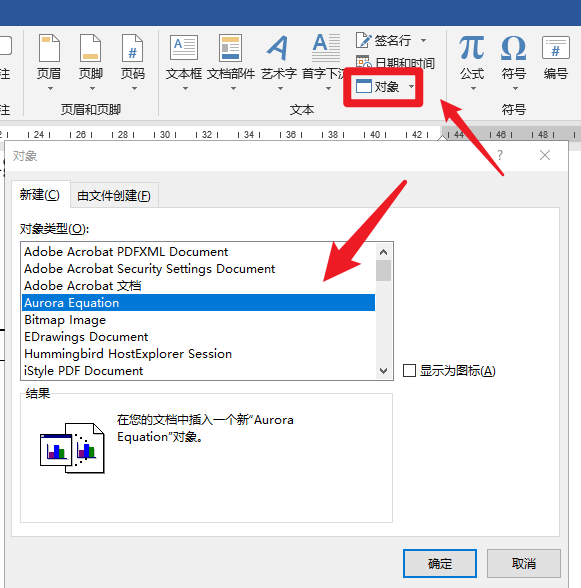下面提供几个示例 示例1
\renewcommand{\thealgorithm}{1}
\begin{algorithm}[H]
\caption{algorithm caption} %算法的名字
\hspace*{0.02in} {\bf Input:} %算法的输入， \hspace*{0.02in}用来控制位置，同时利用 \\ 进行换行
input parameters A, B, C\\
\hspace*{0.02in} {\bf Output:} %算法的结果输出
output result
\begin{algorithmic}
\State some description % \State 后写一般语句
\For{condition} % For 语句，需要和EndFor对应
\State ...
\If{condition} % If 语句，需要和EndIf对应
\State ...
\Else
\State ...
\EndIf
\EndFor
\While{condition} % While语句，需要和EndWhile对应
\State ...
\EndWhile
\State \Return result
\end{algorithmic}
\end{algorithm}

效果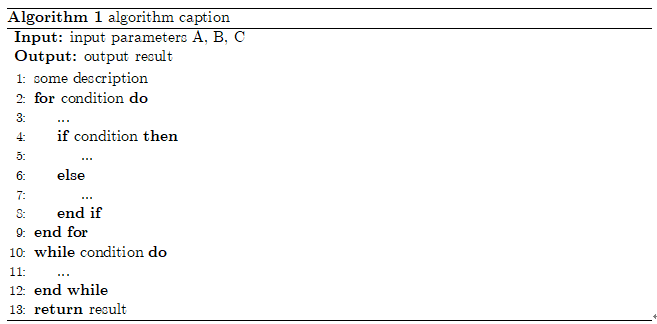示例2
\renewcommand{\thealgorithm}{1}
\begin{algorithm}[H]
\caption{*******************************************}
\label{ABCLFRS}
\begin{algorithmic}
\Require{S,$\lambda$,T,k}
\Ensure{$\mathbf{w}_{222}$}\\
\textbf{initialize}: Set $\mathbf{w}_1 = 0$
\For{$t = 1,2,...,T$}
\State Choose $A_t \subset[m]$
\EndFor
\end{algorithmic}

\end{algorithm}


效果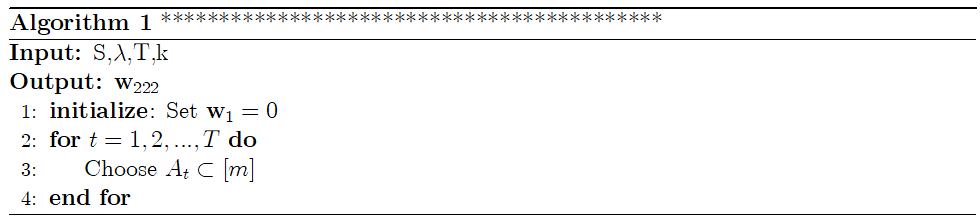示例3
\begin{algorithm}[H]
\caption{algorithm1}
\begin{algorithmic}
\Require
Enter .....;
\Ensure
Outpur......
\State state1......
\State state2......
\State state3......
\While{(a$>$b)}

\State  state4......
\If { c$<$d}
\State state5......
\Else
\State state6......
\EndIf
\State state7......
\EndWhile
\For{aaa}
\State state8......
\EndFor
\end{algorithmic}
\end{algorithm}

效果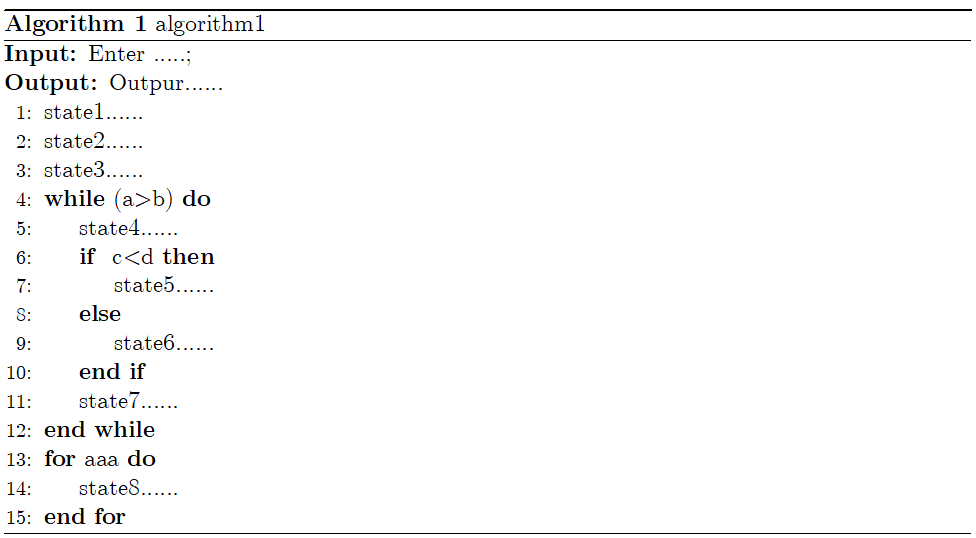参考
此次安装中以下文章提供了帮助，感谢作者！ office中的Aurora公式插件，超好用（含下载安装包） Aurora中出现报错Problems running LaTex，已解决 Aurora problems running latex 的解决 Word2016写论文之——安装Aurora编辑Latex公式及书写伪代码
重点感谢下面几篇！ 【latex】2 使用Aurora与在word中编写伪代码 如何在Word中优雅地插入伪代码 word2016中写出伪代码 如何在WPS/WORD中解决Aurora的运行问题
展开全文aurora
• ## Office之word中插入Latex伪代码

万次阅读 热门讨论 2019-01-24 18:34:49
但有时我们必须要使用word，那么怎么在word插入Latex的伪代码呢？ 下载Aurora.Aurora是一个很好用的公式和伪代码编辑器。 安装后打开word，如果有插件直接在插件里打开，没有的话就在插入-对象-Aurora ...
latex一直是一个高冷的排版工具，写出的伪代码可读性强，样式美观。 但有时我们必须要使用word，那么怎么在word里插入Latex的伪代码呢？
下载Aurora.Aurora是一个很好用的公式和伪代码编辑器。自行搜索下载~安装后打开word，如果有插件直接在插件里打开，没有的话就在插入-对象-Aurora equation打开导入包：Properties

→

\rightarrow

packages粘贴一下的包：
\usepackage{amsmath}
\usepackage{amssymb}
% \usepackage{euler}
\usepackage{amsfonts}
\usepackage{mathrsfs}

\renewcommand{\algorithmicrequire}{\textbf{Input:}}
\renewcommand{\algorithmicensure}{\textbf{Output:}}

\usepackage{multirow}
\usepackage{CJK}
\usepackage{algorithm}
\usepackage{algorithmic}

\providecommand{\abs}{\left\lvert#1\right\rvert}
\providecommand{\norm}{\left\lVert#1\right\rVert}

正文可以复制代码（以下为参考示例）
\renewcommand{\thealgorithm}{1}   %定义算法的编号
\begin{algorithm}[H]
\caption{Doppler Profile Extraction}
\begin{algorithmic}
\REQUIRE{Spectrogram matrix $P$, centre frequency bin $cf$}
\ENSURE{Doppler shift profile $DopShift$}\\
\STATE{colNum=getColumNum($P$);} \\
\STATE{$DopShift$(1:colNum)=$cf$;}  //initialization \\
\FOR{i=1:colNum}
\STATE row=getNonNullRows(col(i)); // get non-null rows of the $i$th col\\
\IF{isNotEmpty(row)}
\STATE{meanValue=mean(row);}\\
\ELSE
\STATE{meanValue\textgreater cf}\\
\STATE{$DopShift$(i)=max(row);}\\
\STATE{$DopShift$(i)=min(row);}\\
\ENDIF
\ENDFOR
\STATE{$DopShift$ = SMA($DopShift$);} // SMA represents smoothed moving average filter\\
\STATE{Return $DopShift$;}
\end{algorithmic}
\end{algorithm}

几点说明：
Aurora的伪代码按照的是\end{algorithmic}包语法，不是\end{algorithm2e}。如果使用2e包写的latex，需要再改一下。具体不同请看上述代码示例或者查看{algorithmic}包介绍, 或者这篇.命令要大写，如STATE;如果出现问题，请仔细检查自己的代码是否和上述示例一致。
得到结果预览如下：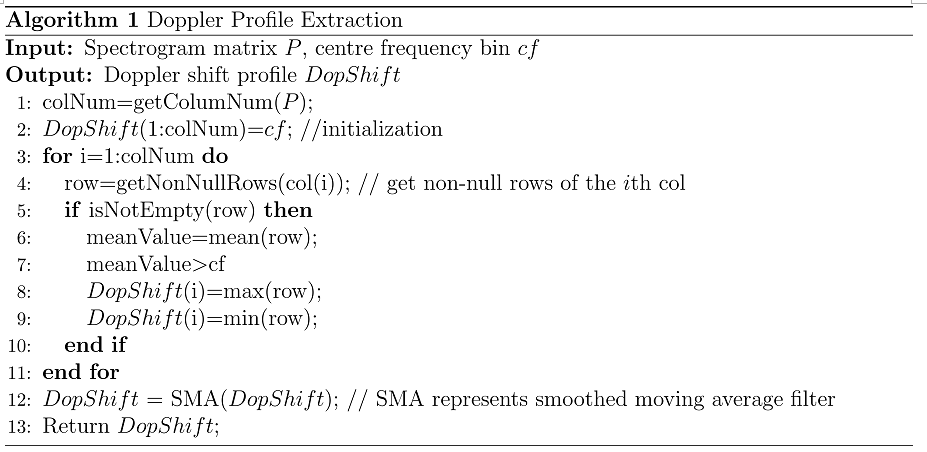展开全文latex matlab
• 写论文的时候，时常需要我们将算法以伪代码的形式展示出来，我们都知道latex可以帮我们生成一个漂亮的伪代码格式，但是在word中我们该如何加入呢？ 可以使用Aurora插件实现。Latex in word 1. 插入算法伪代码 在...
写论文的时候，时常需要我们将算法以伪代码的形式展示出来，我们都知道latex可以帮我们生成一个漂亮的伪代码格式，但是在word中我们该如何加入呢？
可以使用Aurora插件实现。Latex in word
1. 插入算法伪代码
在Word2016中点击插入->对象->选择Aurora Equation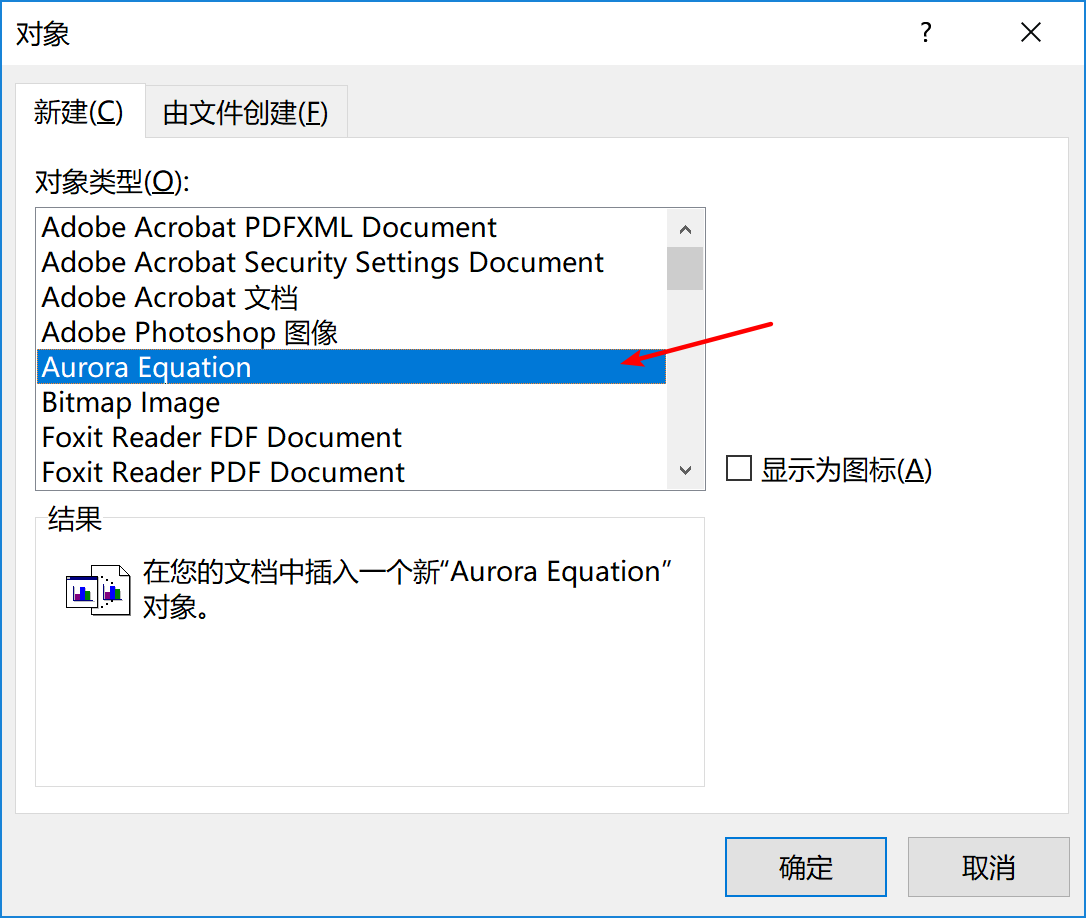此时会打开Aurora公式编辑框，在其中写入：
\renewcommand{\thealgorithm}{1}
\begin{algorithm}[H]
\caption{*******************************************}
\label{ABCLFRS}
\begin{algorithmic}
\Require{S,$\lambda$,T,k}
\Ensure{$\mathbf{w}_{222}$}\\
\textbf{initialize}: Set $\mathbf{w}_1 = 0$
\For{$t = 1,2,...,T$}
\State Choose $A_t \subset[m]$
\EndFor
\end{algorithmic}

\end{algorithm}
并在编辑框中的Properties菜单中的Packages选项卡中填入所用到的包: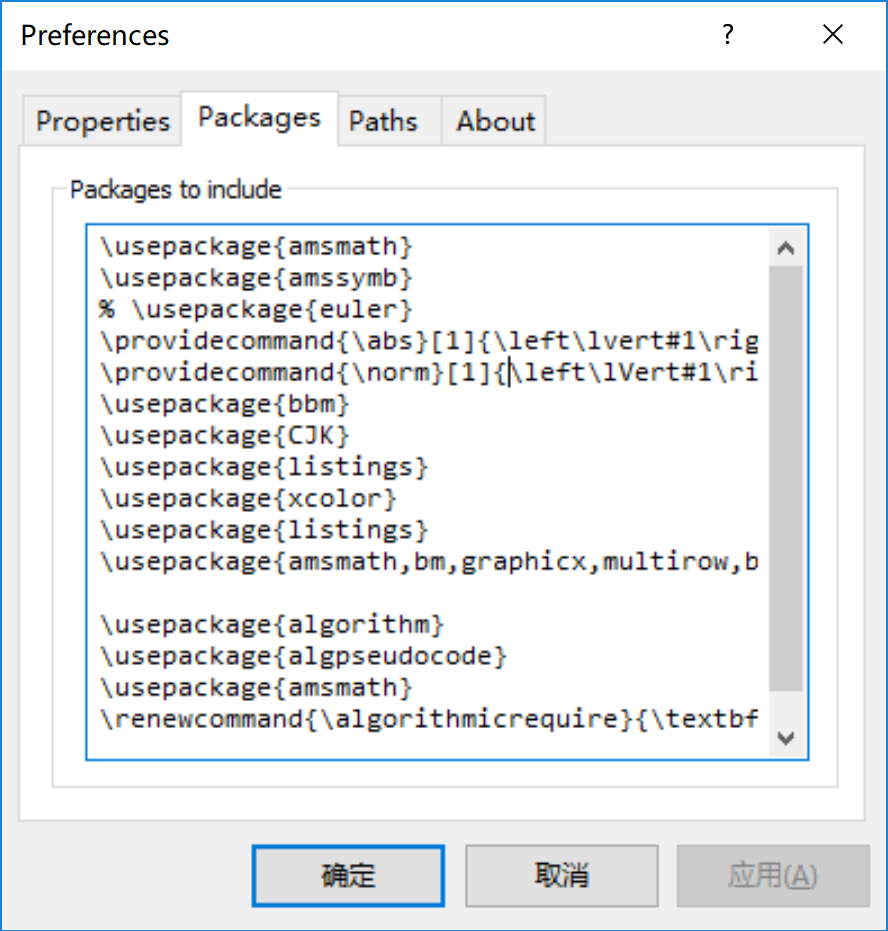\usepackage{amsmath}
\usepackage{amssymb}
% \usepackage{euler}
\providecommand{\abs}{\left\lvert#1\right\rvert}
\providecommand{\norm}{\left\lVert#1\right\rVert}
\usepackage{bbm}
\usepackage{CJK}
\usepackage{listings}
\usepackage{xcolor}
\usepackage{listings}
\usepackage{amsmath,bm,graphicx,multirow,bm,bbm,amssymb,psfrag,algorithm,subfigure,color,mdframed,wasysym,subeqnarray,multicol}

\usepackage{algorithm}
\usepackage{algpseudocode}
\usepackage{amsmath}
\renewcommand{\algorithmicrequire}{\textbf{Input:}}
\renewcommand{\algorithmicensure}{\textbf{Output:}}
最后Refresh一下，就可以得到最终的伪代码了，如下图所示：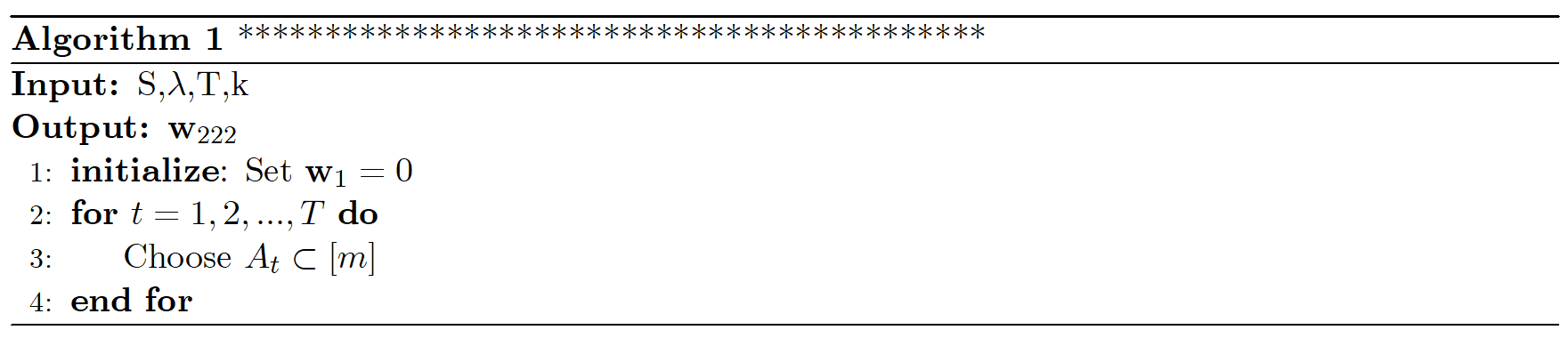2. 插入表格
假设您需要将下面的latex转换到word中，又不想改变其排版方式，可以通过Aurora轻松帮您实现。
推荐一个excel转为latex的excel插件：https://ctan.org/tex-archive/support/excel2latex/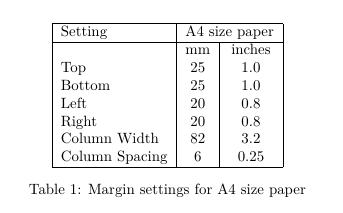\begin{table}
\centering
\begin{tabular}{|l|c|c|}\hline
Setting&\multicolumn{2}{c|}{A4 size paper}\\\hline
&mm&inches\\
Top&25&1.0\\
Bottom&25&1.0\\
Left&20&0.8\\
Right&20&0.8\\
Column Width&82&3.2\\
Column Spacing&6&0.25\\\hline
\end{tabular}
\caption{Margin settings for A4 size paper}
\end{table}
将上述的latex代码Ctrl+c复制，到word中找到Aurora选项卡，选择paste from tex选项即可。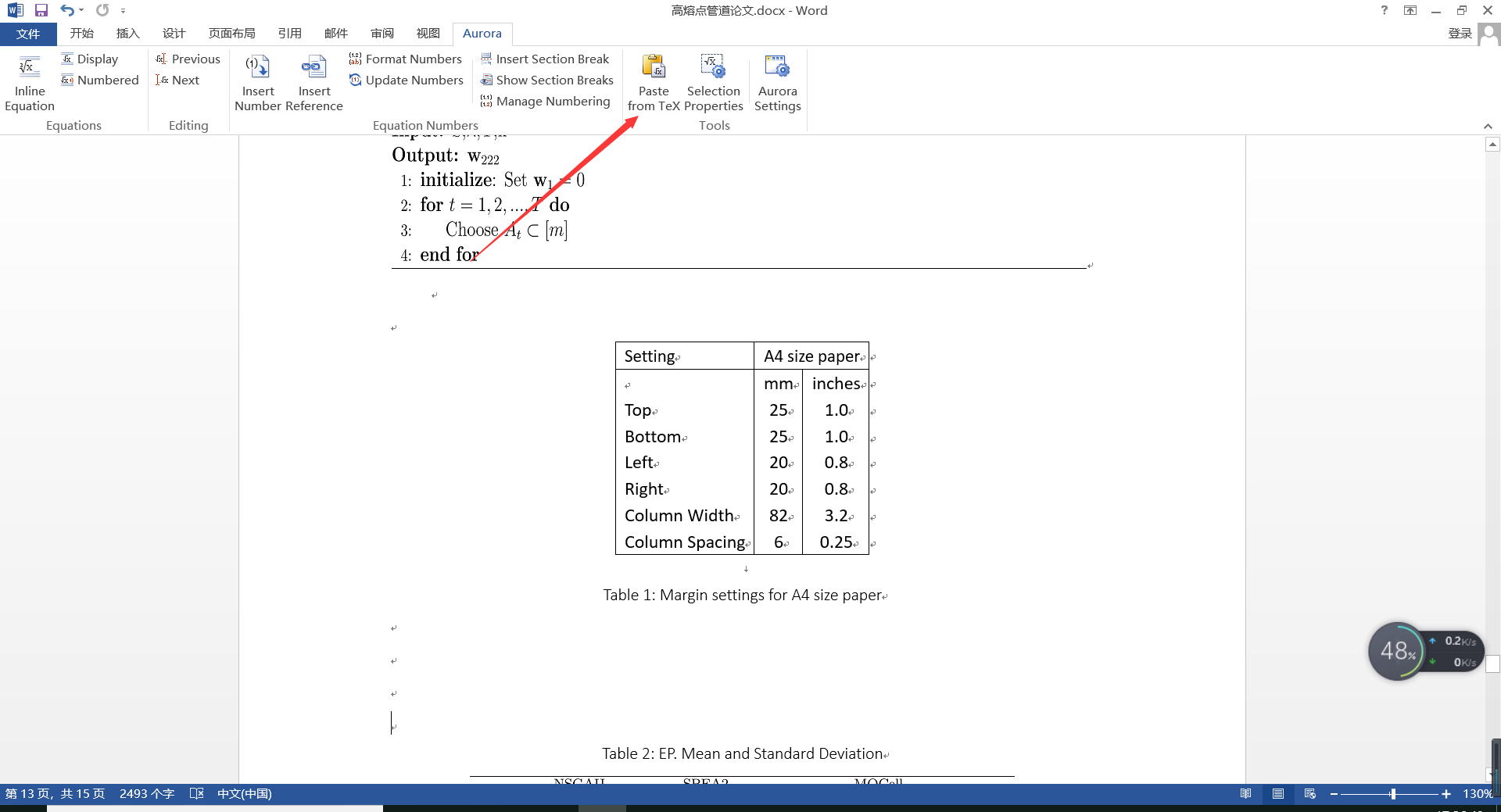3. 插入公式
这里推荐一个软件Mathpix，这个软件可以直接截图，将图片中的公式自动转换为latex代码，这样我们就可以直接像上面的做法一样，将latex代码导入到word中。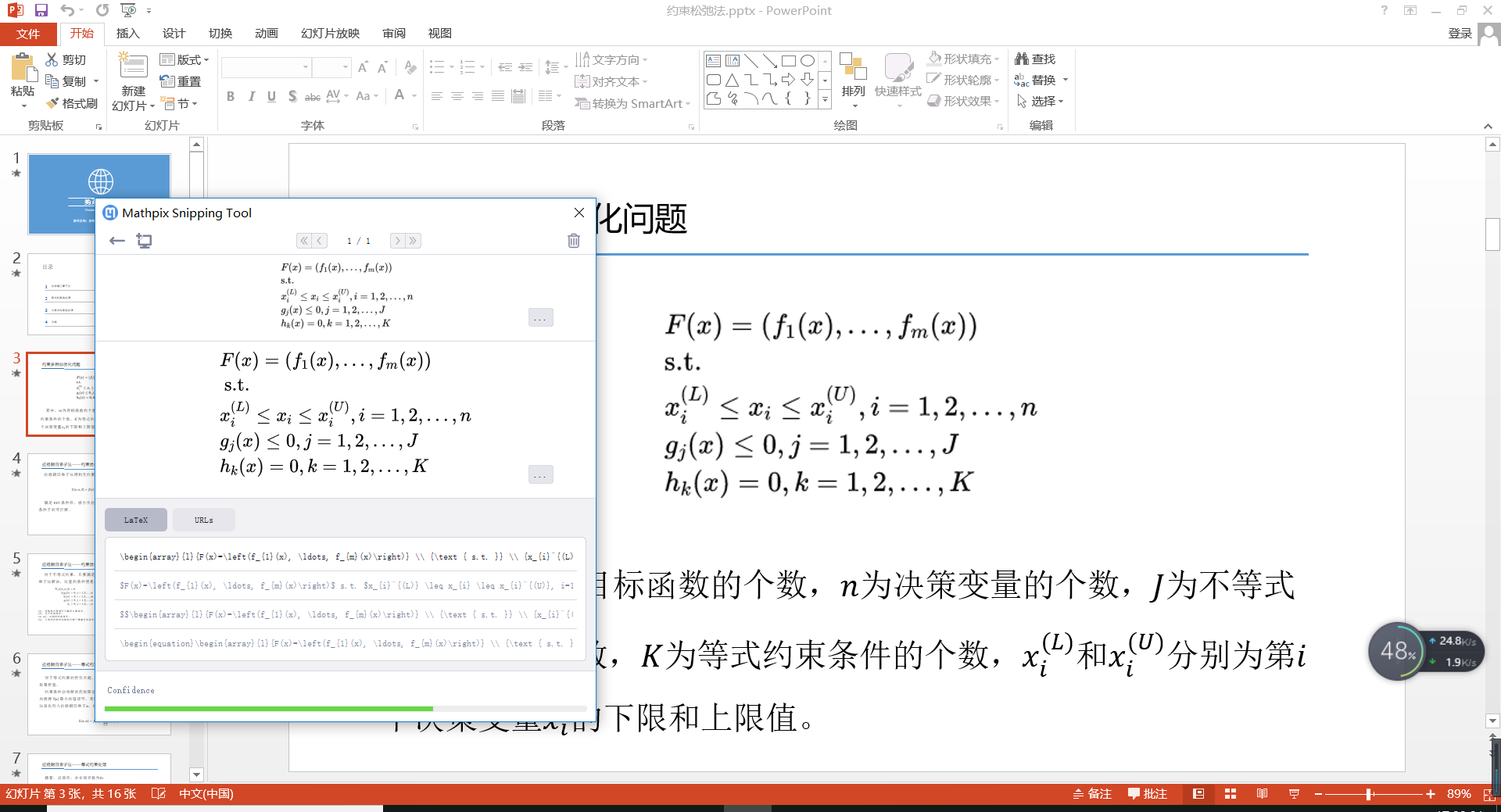导入到word中的效果如下所示：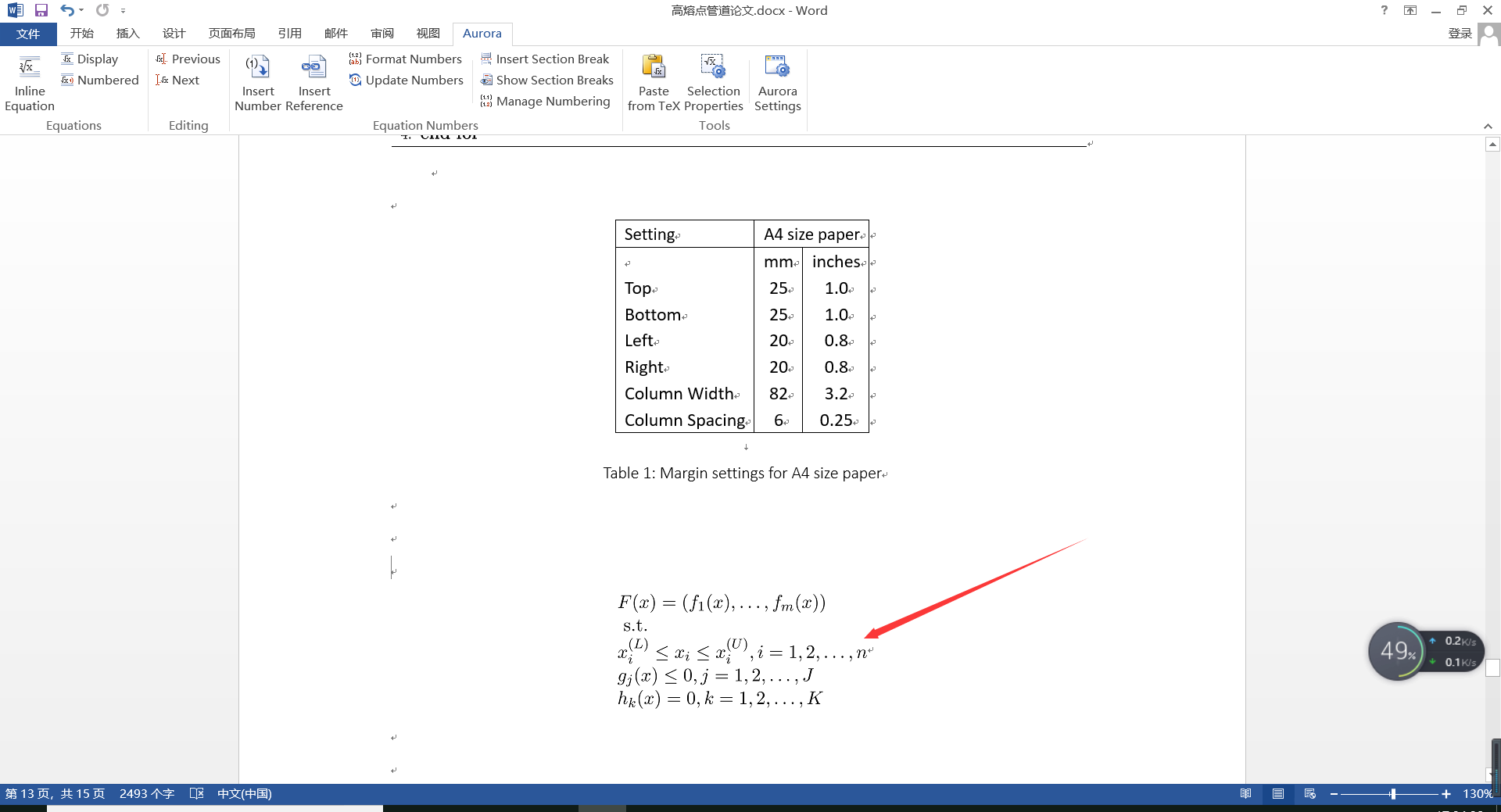展开全文latex
• 使用word时，需要插入latex样式的伪代码，如下所示： 安装后打开word，如果有插件直接在插件里打开，没有的话就在插入-对象-Aurora equation打开。 导入包：Properties→packages粘贴一下的包： \usepackage{...
• 这里主要是想说一下如何在word插入算法的伪代码。 一、使用Aurora插件 二、使用表格doc
• ## 如何在word中插入latex公式和伪代码

万次阅读 多人点赞 2017-12-25 20:42:36
如何在word插入latex公式和伪代码word中可以通过软件Aurora把latex公式直接搬到word里面，超级好用，安装完软件之后，word的工具栏会自动出现该选项。至于伪代码，只要复制，点击工具栏的 Paste from Tex 就可以...latex
• 如何在word中写伪代码 1.下载texlive 参考https://blog.csdn.net/Mikchy/article/details/94448707 2.下载Aurora 下载链接 链接: https://pan.baidu.com/s/1GpTaSq_XG6Jm9WHTcZYkwQ 提取码: 2fxc 复制这段内容后打开...
• 回到Word，就会发现插入伪代码成功！！！ 到这里，就结束了，剩下的工作就是学会使用这种语法，将自己的伪代码放到论文中去！ 如果百度网盘链接失效（我设置了永久有效）或者哪里有问题可以留言。
• 亲测可用，参考链接：https://blog.csdn.net/LIN_SHUQING/article/details/78896623?utm_source=blogxgwz1
• ## WPS插入LaTeX伪代码

万次阅读 2018-05-20 12:03:24
本来一直都是用word写论文，最近需要插入伪代码，网上搜了下可以安装一个插件，叫做 Aurora，具体方法参考百度经验“如何在WPS或word插入伪代码”但是上文没有给参考程序，于是博主直接找的 LaTeX 写伪代码的程序...
• 不过如果我们想把论文投向日益发展不错的国内期刊，那么问题就来了，国内期刊有些要求论文的Word格式，且给出的也是Word模板，如果我们还想保留Latex帅气的算法怎么办，莫慌，可以按如下要求操作： 1.你需要下载一...latex
• -> Ctex -> Settings(Admin) -> Refresh FNDB" 3、安装 Inkspace （将生成的 算法步骤图/伪代码.pdf 转成能导入word的格式）https://inkscape.org/release/inkscape-0.92.4/ 好吧，开始操作~~~ 装好 CTEX 后，打开 ...
• WPS中用Aurora写伪代码 第一步 在Properties->Packages中添加 \usepackage{algorithm} \usepackage{algpseudocode} \usepackage{amsmath} \usepackage{setspace} \renewcommand{\algorithmicrequire}{\textbf{...LaTeX Aurora
• 如果希望在论文中使用 latex 编写伪代码，就像下图一样简洁美观的话，可以考虑继续阅读。 下载安装与配置 Aurora 1. 确保安装好了 latex 前面介绍了安装一个很大的包 latexlive 环境安装与快速上手，这一个步骤...latex
• 前提说明 1、Aurora好像只支持在32位Word中菜单栏显示加载项； 2、所有安装包下载见此处 安装步骤 详情请见：http://www.yuerya.me/post/aurora-|-latex-in-wordAurora
• 最近在工作中，需要在详细设计文档中插入伪代码。尝试编辑了不同的格式，总是觉得代码显示的不美观，因此尝试在网上搜索一些方法，还真有这方面的资料。如下 一、CSDN的博文《如何优雅的在 Microsoft word中插入...
• 图片 别忘了开头插入库（usepackage{graphicx}） 1）插入单张图片（从模板提取代码）： begin{figure}[!t] centering includegraphics[width=2.5in]{luna.jpg} caption{this is a figure demo} label{fig_sim} end{...
• Latex中加入算法伪代码模板 1.加入包，一定放在 \begin{document}之前 \usepackage{algorithm} \usepackage{algpseudocode} \usepackage{amsmath} \renewcommand{\algorithmicrequire}{\textbf{Input:}} \...latex
• 要注意这几点： overleaf开头写的导包语句要放到Aurora的Packages 字体、页面等配置不用写到Packages，在Aurora里可以直接设置，而不用代码 伪代码主体就可以粘到Aurora了，但是没有预期生成伪代码，提示Problem ...latex aurora...